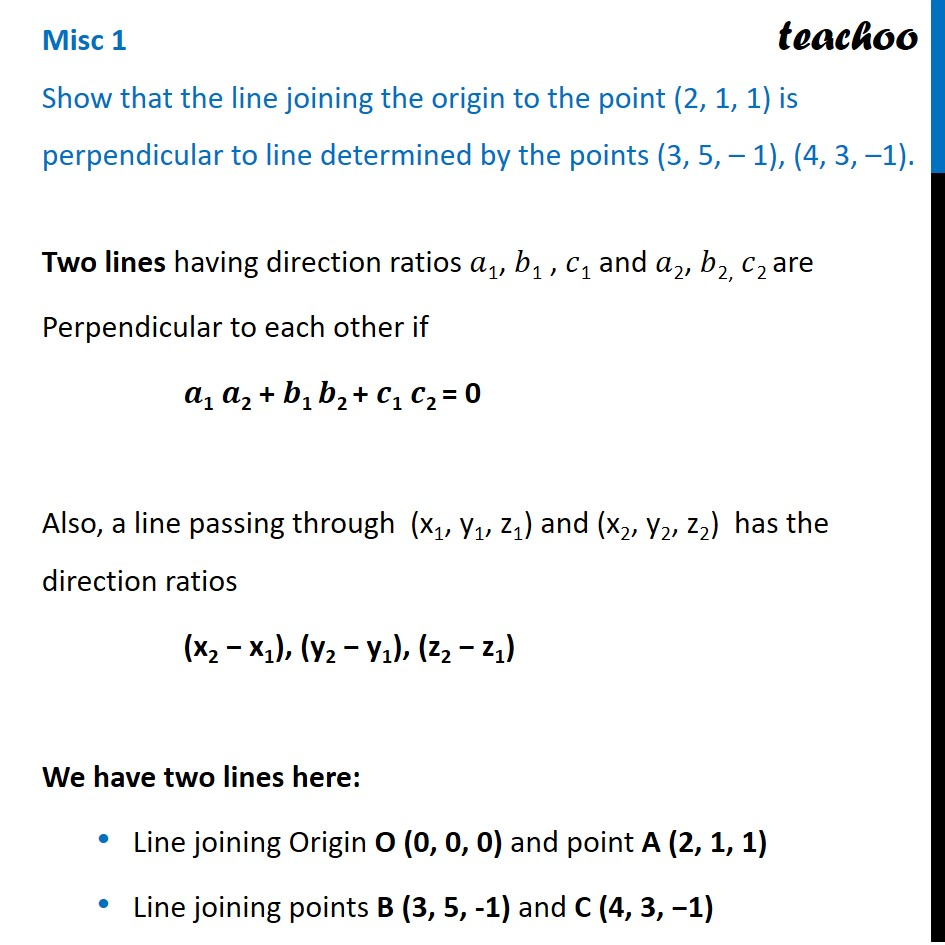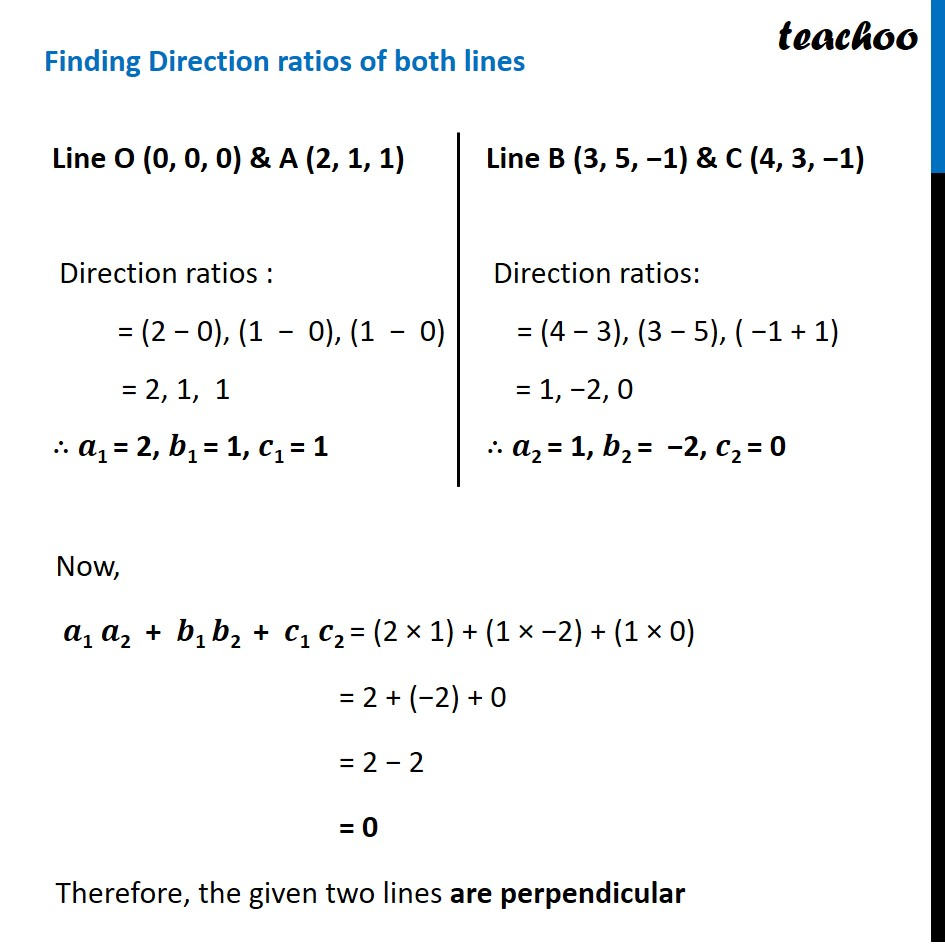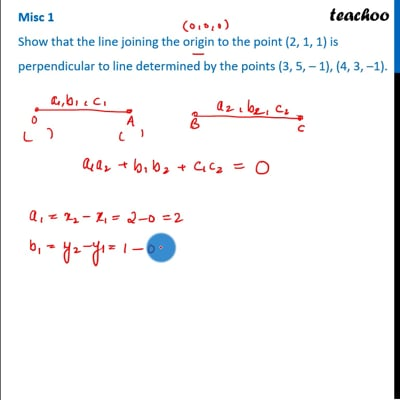Miscellaneous

Chapter 11 Class 12 Three Dimensional Geometry
Serial order wiseThis video is only available for Teachoo black users

Solve all your doubts with Teachoo Black (new monthly pack available now!)

### Transcript

Misc 1 Show that the line joining the origin to the point (2, 1, 1) is perpendicular to line determined by the points (3, 5, – 1), (4, 3, –1).Two lines having direction ratios 𝑎1, 𝑏1 , 𝑐1 and 𝑎2, 𝑏2, 𝑐2 are Perpendicular to each other if 𝒂1 𝒂2 + 𝒃1 𝒃2 + 𝒄1 𝒄2 = 0 Also, a line passing through (x1, y1, z1) and (x2, y2, z2) has the direction ratios (x2 − x1), (y2 − y1), (z2 − z1) We have two lines here: Line joining Origin O (0, 0, 0) and point A (2, 1, 1) Line joining points B (3, 5, -1) and C (4, 3, −1) Finding Direction ratios of both lines Line O (0, 0, 0) & A (2, 1, 1) Direction ratios : = (2 − 0), (1 − 0), (1 − 0) = 2, 1, 1 ∴ 𝒂1 = 2, 𝒃1 = 1, 𝒄1 = 1 Line B (3, 5, −1) & C (4, 3, −1) Direction ratios: = (4 − 3), (3 − 5), ( −1 + 1) = 1, −2, 0 ∴ 𝒂2 = 1, 𝒃2 = −2, 𝒄2 = 0 Now, 𝒂1 𝒂2 + 𝒃1 𝒃2 + 𝒄1 𝒄2 = (2 × 1) + (1 × −2) + (1 × 0) = 2 + (−2) + 0 = 2 − 2 = 0 Therefore, the given two lines are perpendicular